Sept. 24, 2019

# An overview of Face detection

This is the first post of two where I am going to talk about face recognition.

In order to train or use a face recognition system we need to localize the face or faces in the input image, once we have localized the face we align it. Since we are only using the region of interest (in this case the face) we reduce the noise from the background which could affect the recognition. This task is called Face Detection.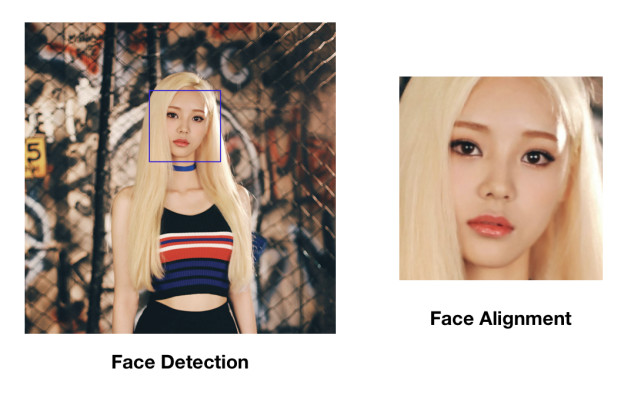There are different ways to localize faces in an image, in this post we will talk about the Multi-task Cascade Convolutional Neural Network and the Haar-Like algorithm.

One popular and fast architecture to localize faces is the MTCNN or Multi-task Cascade Convolutional Neural Network. This architecture consists of three convolutional neural networks which identify faces with high accuracy.

To train this neural network, for each input image we have to build an image pyramid. These are a collection of images which contains the same image in different scales.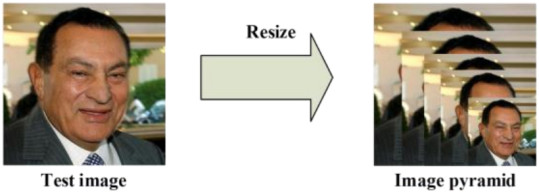Image Pyramid

#### Stage 1. Proposal Network (P-Net)

Using a kernel/filter we take multiple portions of each image in the image pyramid collection of size 12x12 which the P-Net uses as input. The network scans each portion looking for faces.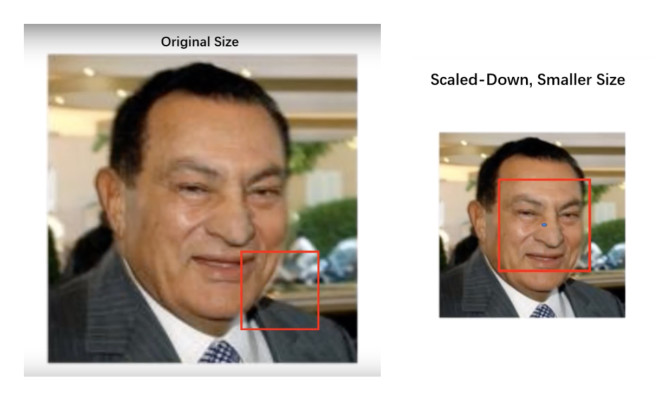Since we are using image pyramid of multiple scales, we can find the face in an image more easily. https://www.youtube.com/watch?v=w4tigQn-7Jw

We will obtain multiple bounding boxes from the P-net and we will have to apply non-maximum suppression to only keep the bounding boxes with a high confidence where the network is confident that a face appears.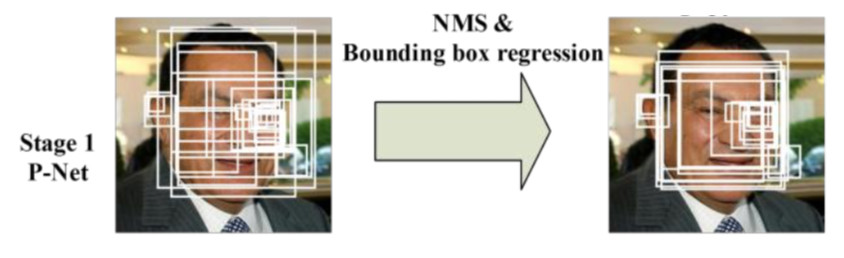Stage 1 (P-Net)

#### Stage 2. Refine Network (R-Net)

The second neural network called Refine Network takes as input all the faces that we kept in the stage 1 and further rejects false candidates. In other words, this network Refine all the bounding boxes.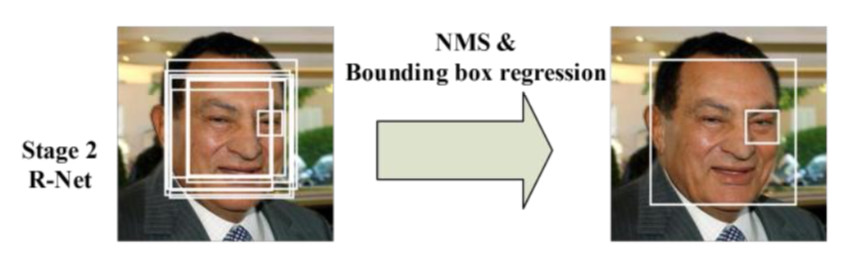Stage 2 (R-Net)

#### Stage 3. Output Network (O-Net)

This networks does a similar job as the refine network but not only outputs the bounding boxes, it also outputs facial landmarks’ positions.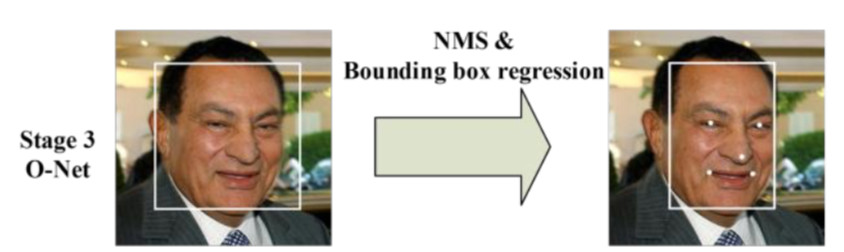Stage 3 (O-Net)

Even Though this big network consists of three convolutional neural networks, we can obtain a real time performance which makes this architecture really good to detect faces. If you want to know more about it you can read the paper in this link.

The main idea behind this technique is boosting. Boosting is an ensamble that uses multiple models which are organized into a hierarchy or cascade. Each model learns the weaknesses of the previous model. Due to this, the first models learn simple features and the last models learn more complex features.

The cascare classifier uses the Haar-Like features algorithm to obtain features from the face. This algorithm takes into account that most human faces share properties like the eyes region which is darker than the nose region.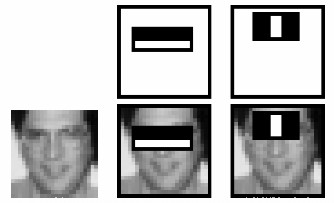Perhaps this is the easiest way to detect faces in images since we can use openCV which contains this algorithm.

With the following code we can detect faces in images using the cascade classifier. We need to install open cv2, matplotlib and download this file

``````
import cv2

import numpy as np

import matplotlib.pyplot as plt

gray_img = gray = cv2.cvtColor (img, cv2.COLOR_BGR2GRAY)

faces = face_classifier.detectMultiScale(gray_img, scaleFactor=1.1, minNeighbors=5, flags=cv2.CASCADE_SCALE_IMAGE)

for (x, y, width, height) in faces:

cv2.rectangle(img, (x, y), (x + width, y + height), (220, 20, 60), 3)

plt.figure(figsize = (10, 6))

plt.imshow(img)

plt.show()

``````